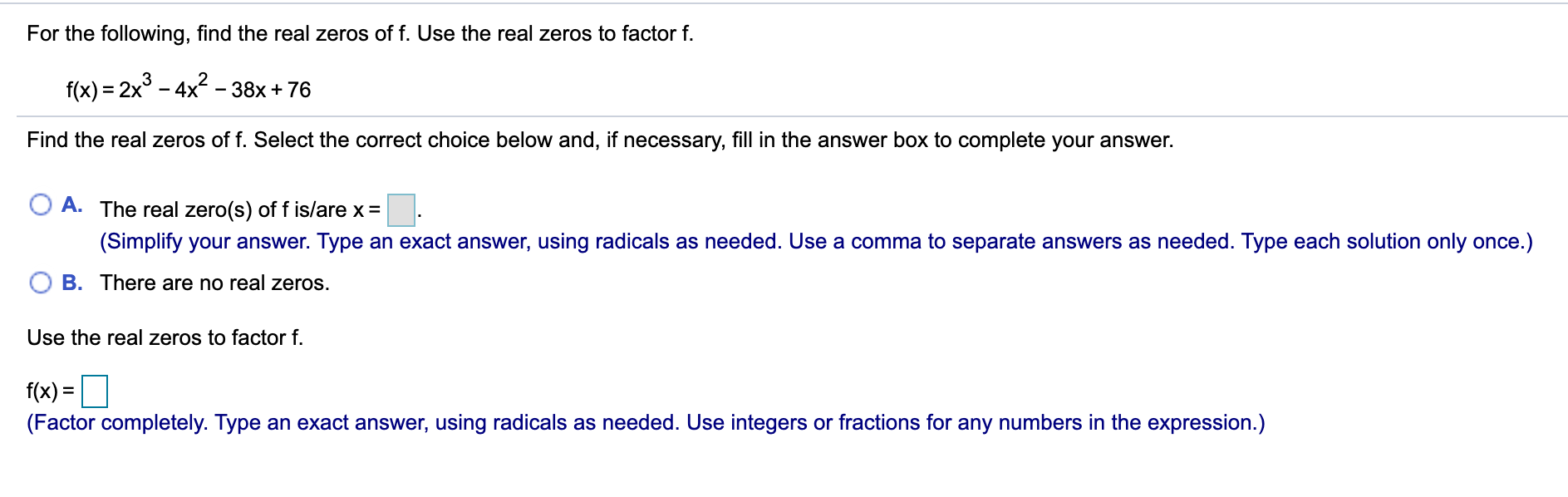# For the following, find the real zeros of f. Use the real zeros to factor f. f(x) = 2x° - 4x - 38x + 76 %3D Find the real zeros of f. Select the correct choice below and, if necessary, fill in the answer box to complete your answer. A. The real zero(s) of f is/are x = (Simplify your answer. Type an exact answer, using radicals as needed. Use a comma to separate answers as needed. Type each solution only once.) B. There are no real zeros. Use the real zeros to factor f. f(x) = (Factor completely. Type an exact answer, using radicals as needed. Use integers or fractions for any numbers in the expression.)

Questionhelp_outlineImage TranscriptioncloseFor the following, find the real zeros of f. Use the real zeros to factor f. f(x) = 2x° - 4x - 38x + 76 %3D Find the real zeros of f. Select the correct choice below and, if necessary, fill in the answer box to complete your answer. A. The real zero(s) of f is/are x = (Simplify your answer. Type an exact answer, using radicals as needed. Use a comma to separate answers as needed. Type each solution only once.) B. There are no real zeros. Use the real zeros to factor f. f(x) = (Factor completely. Type an exact answer, using radicals as needed. Use integers or fractions for any numbers in the expression.) fullscreen